Demonstration line drawing code - WoWInterface
 01-13-06, 01:59 AM #1 Iriel Super Moderator Demonstration line drawing code Since a couple of people expressed interest in how to do this, I've put together some code which shows how you can use SetTexCoord in 1.9 to draw lines using Texture objects. The code can be found at http://www.vigilance-committee.org/wow/downloads/ LineDrawTest-0.1-10900.zip It's got a silly bouncing line demo in it, and you can look at the code (Though i'm going to include that below). Here's how it works (logically) 1. A line between two points can be thought of as a rectangle of a desired width and length, positioned (translated and rotated) to run from the first point to the second. 2. I take a square image, scale it in each direction to be the right shape, and rotate it to have the right orientation, then put it into a Texture object in the right place on the screen. In actuality the square image has to have a transparent border of pixels, so there's a slight bit of adjustment for that, but it's the basic idea. Put into code using a 256x256 texture (254x254 solid, with a 1 pixel transparent border) it's: Code: ```local LINEFACTOR = 256/254; -- Multiplying factor for texture coordinates local LINEFACTOR_2 = LINEFACTOR / 2; -- Half o that -- T - Texture -- C - Canvas Frame (for anchoring) -- sx,sy - Coordinate of start of line -- ex,ey - Coordinate of end of line -- w - Width of line -- relPoint - Relative point on canvas to interpret coords (Default BOTTOMLEFT) local function DrawLine(T, C, sx, sy, ex, ey, w, relPoint) if (not relPoint) then relPoint = "BOTTOMLEFT"; end -- Determine dimensions and center point of line local dx,dy = ex - sx, ey - sy; local cx,cy = (sx + ex) / 2, (sy + ey) / 2; -- Normalize direction if necessary if (dx < 0) then dx,dy = -dx,-dy; end -- Calculate actual length of line local l = sqrt((dx * dx) + (dy * dy)); -- Quick escape if it's zero length if (l == 0) then T:SetTexCoord(0,0,0,0,0,0,0,0); T:SetPoint("BOTTOMLEFT", C, relPoint, cx,cy); T:SetPoint("TOPRIGHT", C, relPoint, cx,cy); return; end -- Sin and Cosine of rotation, and combination (for later) local s,c = -dy / l, dx / l; local sc = s * c; -- Calculate bounding box size and texture coordinates local Bwid, Bhgt, BLx, BLy, TLx, TLy, TRx, TRy, BRx, BRy; if (dy >= 0) then Bwid = ((l * c) - (w * s)) * LINEFACTOR_2; Bhgt = ((w * c) - (l * s)) * LINEFACTOR_2; BLx, BLy, BRy = (w / l) * sc, s * s, (l / w) * sc; BRx, TLx, TLy, TRx = 1 - BLy, BLy, 1 - BRy, 1 - BLx; TRy = BRx; else Bwid = ((l * c) + (w * s)) * LINEFACTOR_2; Bhgt = ((w * c) + (l * s)) * LINEFACTOR_2; BLx, BLy, BRx = s * s, -(l / w) * sc, 1 + (w / l) * sc; BRy, TLx, TLy, TRy = BLx, 1 - BRx, 1 - BLx, 1 - BLy; TRx = TLy; end -- Set texture coordinates and anchors T:SetTexCoord(TLx, TLy, BLx, BLy, TRx, TRy, BRx, BRy); T:SetPoint("BOTTOMLEFT", C, relPoint, cx - Bwid, cy - Bhgt); T:SetPoint("TOPRIGHT", C, relPoint, cx + Bwid, cy + Bhgt); end``` There's a notes.txt file inside the zip above with the derivation of those cryptic values 8-) Hopefully someone out there finds this useful.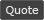WoWInterface » Demonstration line drawing code

 Thread Tools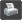Show Printable Version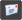Email this Page Display Modes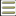Linear Mode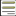Switch to Hybrid Mode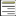Switch to Threaded Mode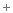Posting Rules You may not post new threads You may not post replies You may not post attachments You may not edit your posts vB code is On Smilies are On [IMG] code is On HTML code is Off